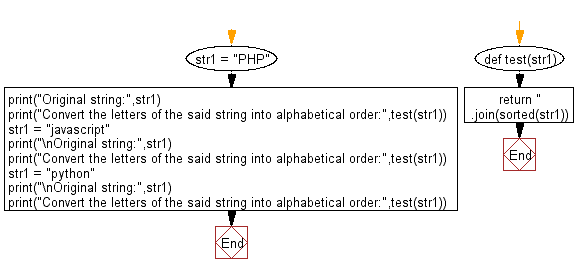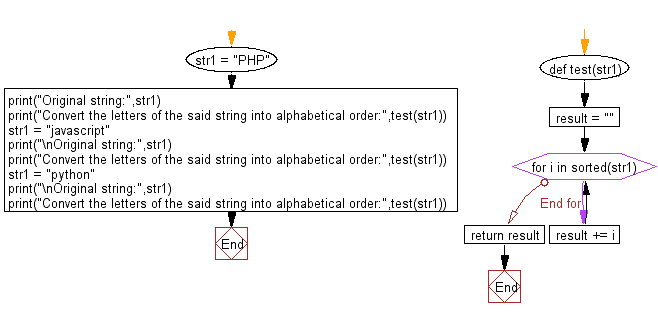﻿ Python: Convert the letters of a given string into alphabetical order - w3resource# Python: Convert the letters of a given string into alphabetical order

## Python Basic - 1: Exercise-126 with Solution

Write a Python program to convert the letters of a given string (same case-upper/lower) into alphabetical order.

Sample Solution-1:

Python Code:

``````def test(str1):
return ''.join(sorted(str1))

str1 = "PHP"
print("Original string:",str1)
print("Convert the letters of the said string into alphabetical order:",test(str1))
str1 = "javascript"
print("\nOriginal string:",str1)
print("Convert the letters of the said string into alphabetical order:",test(str1))
str1 = "python"
print("\nOriginal string:",str1)
print("Convert the letters of the said string into alphabetical order:",test(str1))
``````

Sample Output:

```Original string: PHP
Convert the letters of the said string into alphabetical order: HPP

Original string: javascript
Convert the letters of the said string into alphabetical order: aacijprstv

Original string: python
Convert the letters of the said string into alphabetical order: hnopty
```

Flowchart:Sample Solution-2:

Python Code:

``````def test(str1):
result = ""
for i in sorted(str1):
result += i
return result

str1 = "PHP"
print("Original string:",str1)
print("Convert the letters of the said string into alphabetical order:",test(str1))
str1 = "javascript"
print("\nOriginal string:",str1)
print("Convert the letters of the said string into alphabetical order:",test(str1))
str1 = "python"
print("\nOriginal string:",str1)
print("Convert the letters of the said string into alphabetical order:",test(str1))
``````

Sample Output:

```Original string: PHP
Convert the letters of the said string into alphabetical order: HPP

Original string: javascript
Convert the letters of the said string into alphabetical order: aacijprstv

Original string: python
Convert the letters of the said string into alphabetical order: hnopty
```

Flowchart:Python Code Editor:

Have another way to solve this solution? Contribute your code (and comments) through Disqus.

What is the difficulty level of this exercise?

Test your Programming skills with w3resource's quiz.

﻿

## Python: Tips of the Day

What is the difference between Python's list methods append and extend?

append: Appends object at the end.

```x = [1, 2, 3]
x.append([4, 5])
print (x)
```

Output:

```[1, 2, 3, [4, 5]]
```

extend: Extends list by appending elements from the iterable.

```x = [1, 2, 3]
x.extend([4, 5])
print (x)
```

Output:

```[1, 2, 3, 4, 5]
```

Ref: https://bit.ly/2AZ6ZFq